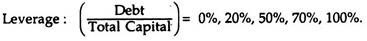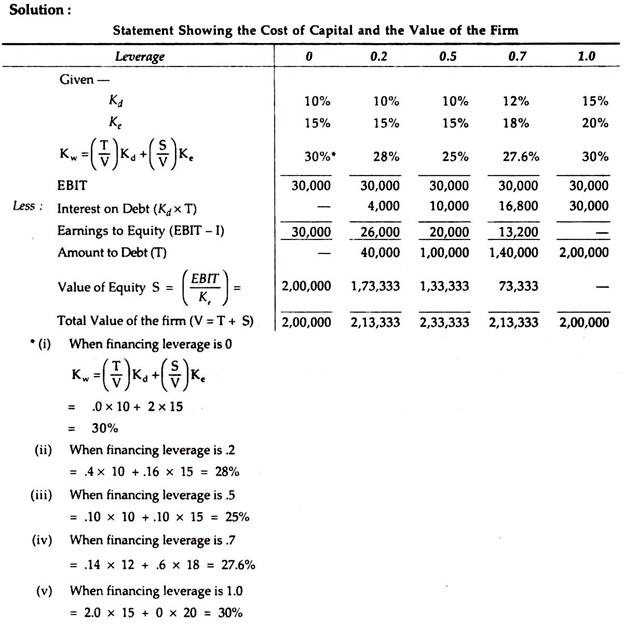It is accepted by all that the judicious use of debt will increase the value of the firm and reduce the cost of capital. So, the optimum capital structure is the point at which the value of the firm is highest and the cost of capital is at its lowest point. Practically, this approach encompasses all the ground between the Net Income Approach and the Net Operating Income Approach, i.e., it may be called Intermediate Approach.

The traditional approach explains that up to a certain point, debt-equity mix will cause the market value of the firm to rise and the cost of capital to decline. But after attaining the optimum level, any additional debt will cause to decrease the market value and to increase the cost of capital.

In other words, after attaining the optimum level, any additional debt taken will offset the use of cheaper debt capital since the average cost of capital will increase along with a corresponding increase in the average cost of debt capital.

Thus, the basic proposition of this approach are

(a) The cost of debt capital, Kd, remains constant more or less up to a certain level and thereafter rises.

(b) The cost of equity capital Ke, remains constant more or less or rises gradually up to a certain level and thereafter increases rapidly.

(c) The average cost of capital, Kw, decreases up to a certain level remains unchanged more or less and thereafter rises after attaining a certain level.

The traditional approach can graphically be represented under taking the data from the previous illustrationIt is found from the above that the average cost curve is U-shaped. That is, at this stage the cost of capital would be minimum which is expressed by the letter ‘A’ in the graph. If we draw a perpendicular to the X-axis, the same will indicate the optimum capital structure for the firm.

Thus, the traditional position implies that the cost of capital is not independent of the capital structure of the firm and that there is an optimal capital structure. At that optimal structure, the marginal real cost of debt (explicit and implicit) is the same as the marginal real cost of equity in equilibrium.

For degree of leverage before that point, the marginal real cost of debt is less than that of equity beyond that point the marginal real cost of debt exceeds that of equity.

Illustration:Calculate the cost of capital and the value of the firm under each of the following alternative degrees of leverage and comment on them:Thus, from the above table, it becomes quite clear the cost of capital is lowest (at 25%) and the value of the firm is the highest (at Rs. 2,33,333) when debt-equity mix is (1,00,000 : 1,00,000 or 1: 1). Hence, optimum capital structure in this case is considered as Equity Capital (Rs. 1,00,000) and Debt Capital (Rs. 1,00,000) which bring the lowest overall cost of capital followed by the highest value of the firm.

### Variations on the Traditional Theory

This theory underlines between the Net Income Approach and the Net Operating Income Approach. Thus, there are some distinct variations in this theory. Some followers of the traditional school of thought suggest that Ke does not practically rise till some critical conditions arise. Only after attaining that level the investors apprehend the increasing financial risk and penalise the market price of the shares. This variation expresses that a firm can have lower cost of capital with the initial use of leverage significantly.

This variation in Traditional Approach is depicted as:Other followers e.g., Solomon, are of opinion the Ke is being saucer-shaped along with a horizontal middle range. It explains that optimum capital structure has a range where the cost of capital is rather minimized and where the total value of the firm is maximized. Under the circumstances a change in leverage has, practically, no effect on the total firm’s value. So, this approach grants some sort of variation in the optimal capital structure for various firms under debt-equity mix.

Such variation can be depicted in the form of graphical representation:## 2 thoughts on “Traditional Approach”

error: Content is protected !!